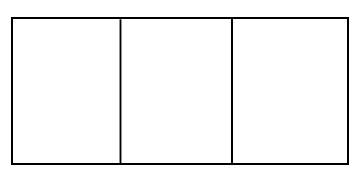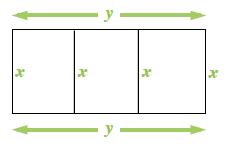### Home > PC3 > Chapter 12 > Lesson 12.2.1 > Problem12-107

12-107.A rectangular pen is to be constructed with three sections as shown in the diagram at right. If the total amount of fencing available is $500$ feet, what is the maximum area that can be enclosed by the fence?

Label the diagram.

Write an equation for the perimeter and the available $500$ feet of fencing. Solve for $y$.Write an equation for the area in terms of $x$ and $y$.

Substitute the expression for $y$ from the perimeter equation into the area equation.

Use your calculator to graph the area function.